# Asg 3: Discrete wavelet transform (DWT)

## Assignment

Implement the Discrete wavelet transform with command-line arguments to select the pyramidal level, e.g., % ./dwt -l 0  would give a 1-level decomposition, while % ./dwt -l 9  would give a full-level $$log_2(512)$$ decomposition of a $$512 \times 512$$ original.

## Suggestions (e.g., for C implementation)

1. This assignment relies on an unrolled recursive implementation of the DWT. If you keep the scaled image within the upper-left quadrant, then your loop just operates on this section of the 2D array, reducing the row and column extent by half each time (see example images below).
2. This assignment also requires the slightly more complicated inverse DWT implementation to make sure that you can reconstruct the image successfully.
3. Any kind of compression or edge or corner detection you want to implement is extra–the simplest thing to do is to decimate wavelet coefficients at some threshold.
4. The Haar wavelets are likely the easiest to implement.

## Further Suggestions

1. For debugging a good idea is to write out and look at the wavelet image itself–because of the range of values, however, (wavelet coefficients are near zero) the output image may look strange. Typically normalizing the (float) values to range $$[0, 1]$$ prior to output is what is required.
2. The main() funcrtion can look something like this (with level hardcoded in this case): int main(int argc,char **argv) { int levels=0; PGM *gimg=NULL, *nimg=NULL; PPM *cimg=NULL; // allocte image of given size cimg = ppm_read("mandrill.ppm"); // convert to grey gimg = ppm_togrey(cimg); // perform the DWT transform to given levels, 0 will do 1 level, // ilog2(img->rows)-1 should do the full DWT, assuming img is square and POT //levels = pgm_levels(gimg); pgm_dwt2D(gimg,levels); pgm_write(gimg,"dgrey.pgm"); // copy DWT image and normalize, for visualization purposes nimg = pgm_copy(gimg); pgm_normalize(nimg); pgm_write(nimg,"ngrey.pgm"); // perform the inverse DWT, should get original image pgm_idwt2D(gimg,levels); // output to file pgm_write(gimg,"grey.pgm"); // free image by sending pointer to pointer to ppm_free function ppm_free(&cimg); pgm_free(&gimg); pgm_free(&nimg); return 0; } 

## Example Program Input & Output

% ./dwt -l 0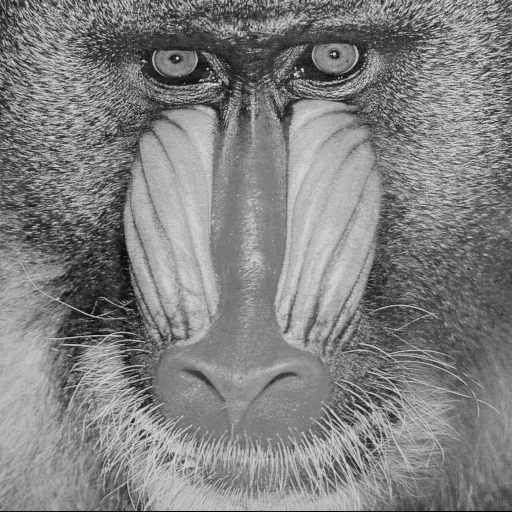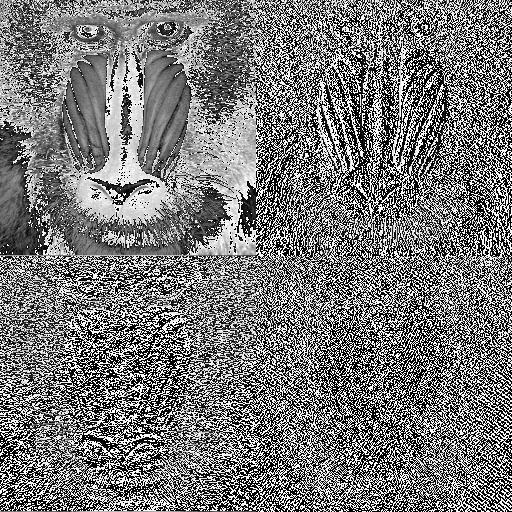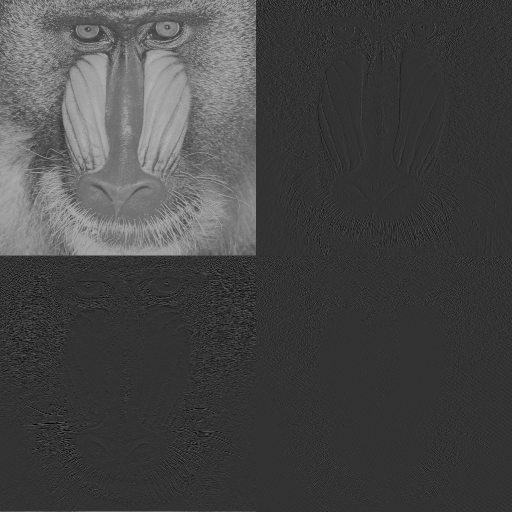% ./dwt -l 1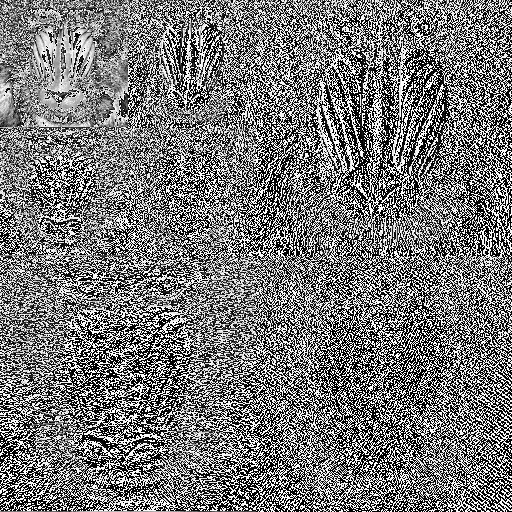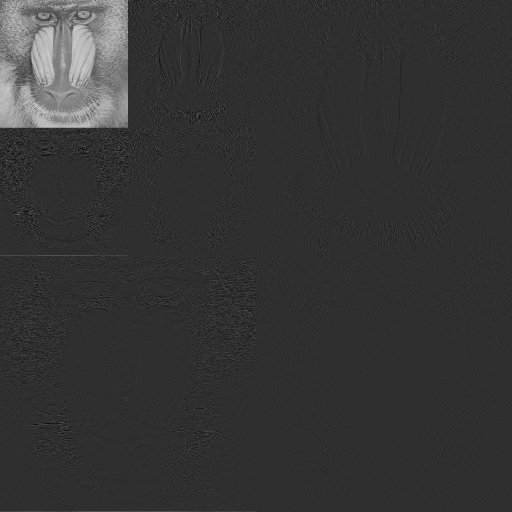## Supplemental

1. If you call your source code file main.c here is a Makefile that you can use to try to compile the project: CC = gcc INCLUDE = -I. CFLAGS = -g LDFLAGS = -L. -L/usr/lib LDLIBS = -lc -lm .c.o: $(CC)$(INCLUDE) $(CFLAGS) -c -o$@ $< OBJECTS = \ pgm.o \ ppm.o \ misc.o \ trig.o \ arrays.o \ wavelets.o \ wtfilter.o \ wt2d.o all: main main: main.o main.c$(CC) $(CFLAGS)$(INCLUDE) -o $@$@.o $(OBJECTS)$(LDFLAGS) \$(LDLIBS) main.o: main.c clean: rm -f *.o rm -rf hotel  Note that each line underneath the targets is indented by a tab, not just spaces, this is important!

## Turn in

Turn in all of your code, in one tar.gz archive of your asg##/ directory, including:
1. A README file containing
1. Course id--section no
2. Name
3. Brief solution description (e.g., program design, description of algorithm, etc., however appropriate).
4. Lessons learned, identified interesting features of your program
5. Any special usage instructions
2. Makefile
3. source code (.h headers and .c source)
4. object code (do a make clean before tar)

## How to hand in

See handin notes